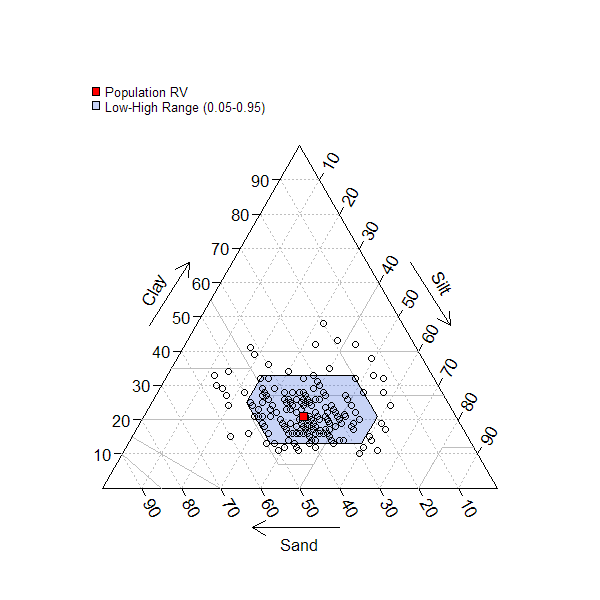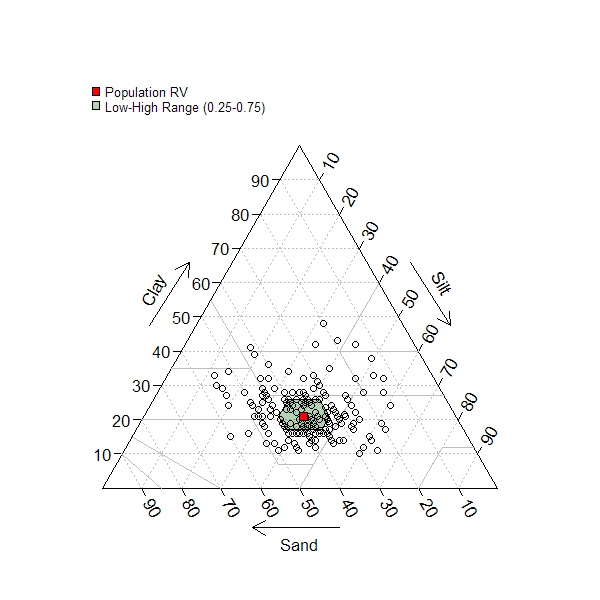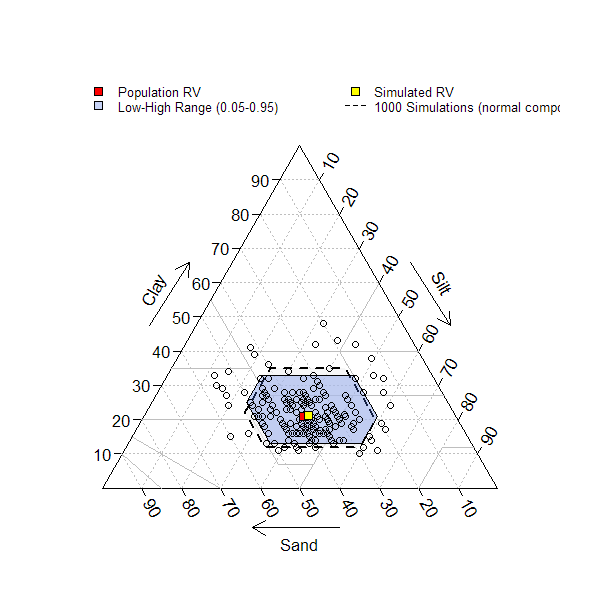texture.triangle.low.rv.high {aqp} R Documentation

## Soil Texture Low-RV-High as Defined by Quantiles

### Description

This function accepts soil texture components (sand, silt, and clay percentages) and plots a soil texture triangle with a "representative value" (point) and low-high region (polygon) defined by quantiles. Marginal quantiles of sand, silt, and clay are used to define the boundary of a low-high region that encloses a severall likely soil texture classes based on the values in `ssc`. The defualt settings place the RV symbol at the texture defined by marginal medians of sand, silt, and clay. The default low-high region is defined by the 5th and 95th marginal percentiles of sand, silt, and clay.

### Usage

```texture.triangle.low.rv.high(ssc, p=c(0.05, 0.5, 0.95), delta=1,
pop.rv.col='red', range.col='RoyalBlue', range.alpha=75,
sim=FALSE, sim.n=1000, sim.rv.col='yellow', sim.col=grey(0.95),
sim.alpha=150, legend.cex=0.75, ...)
```

### Arguments

 `ssc` a matrix-like object with columns: 'sand', 'silt', 'clay', values are percentages that should add to 100. `p` percentiles defining 'low', 'representative value', and 'high' `delta` step-size used to form low-high region `pop.rv.col` the symbol color used to denote the population representative value on the texture triangle `range.col` color of the polygon enclosing the low-high region `range.alpha` transparency of the low-high range polygon (0-255) `sim` optional simulation of low-rv-high values based on a composition drawn from normal distributions, this requires the 'compositions' package `sim.n` number of simulated sand, silt, and clay values `sim.rv.col` the symbol color used to denote the simulated representative value on the texture triangle `sim.col` color of the simulated low-high range polygon `sim.alpha` transparency of the simulated low-high range polygon (0-255) `legend.cex` scaling factor for legend `...` further arguments passed to `triax.points`

### Details

Simulated sand, silt, and clay values are based on sampling from a normal distribution as performed by `rnorm.acomp` in the 'comppositions' package. The mean vector of the sand, silt, and clay values, along with covariance matrix derived from `ssc` are used to parametrize sampling.

### Value

A high-level plot as generated by `soil.texture`.

### Note

Simulation of samd, silt, and clay values requires the 'compositions' package.

### Author(s)

D.E. Beaudette

`triax.points, soil.texture`

### Examples

``````# sample data
data(loafercreek, package='soilDB')

# extract sand, silt, clay proportions
x <- na.omit(data.frame(sand=loafercreek\$sand, silt=loafercreek\$silt, clay=loafercreek\$clay))

# test out the function
texture.triangle.low.rv.high(x, p=c(0.05, 0.5, 0.95))
````````````texture.triangle.low.rv.high(x, p=c(0.25, 0.5, 0.75), range.col='darkgreen')
````````````# simulate compositional data from source data
if(require(compositions)) {
# add simulated low-rv-high
texture.triangle.low.rv.high(x, p=c(0.05, 0.5, 0.95), sim=TRUE)
}
``````[Package aqp version 1.9.1 Index]